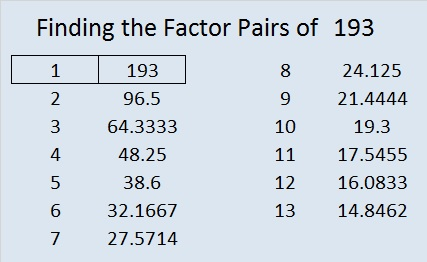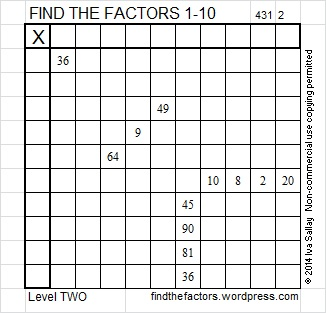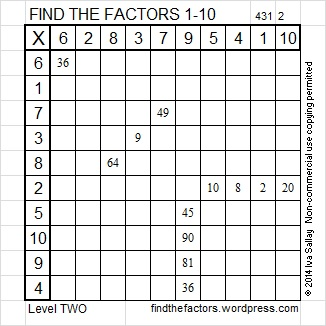# 193 and Level 2

• 193 is a prime number.
• Prime factorization: 193 is prime.
• The exponent of prime number 193 is 1. Adding 1 to that exponent we get (1 + 1) = 2. Therefore 193 has exactly 2 factors.
• Factors of 193: 1, 193
• Factor pairs: 193 = 1 x 193
• 193 has no square factors that allow its square root to be simplified. √193 ≈ 13.89244How do we know that 193 is a prime number? If 193 were not a prime number, then it would be divisible by at least one prime number less than or equal to √193 ≈ 13.9. Since 193 cannot be divided evenly by 2, 3, 5, 7, 11, or 13, we know that 193 is a prime number.Excel file of puzzles and previous week’s factor solutions: 10 Factors 2014-08-04